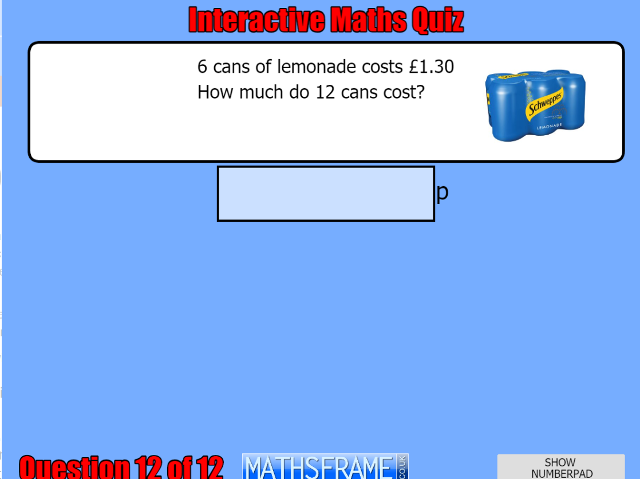## Y4 Number - Multiplication and Division - Interactive Quiz

### Related Worksheets

From 1st January 2020 this game will only be available to subscribers. A single-user games licence costs only £10 per year. You can register here.

An interactive quiz which tests understanding of all the Multiplication and Division objectives in the Year 4 curriculum. Choose one objective or multiple objectives. You can save, or print, your test results as a pdf at the end of the quiz. Ideal for formative or summative assessment.

Alternatively, you can use the Interactive Maths Quiz which includes objectives from all strands of the Year 4 curriculum.

This quiz tests the following objectives:

• recall multiplication and division facts for multiplication tables up to 12 × 12
• use place value, known and derived facts to multiply and divide mentally, including: multiplying by 0 and 1; dividing by 1; multiplying together 3 numbers
• recognise and use factor pairs and commutativity in mental calculations
• multiply two-digit and three-digit numbers by a one-digit number using formal written layout
• solve problems involving multiplying and adding, including using the distributive law to multiply two-digit numbers by 1 digit, integer scaling problems and harder correspondence problems such as n objects are connected to m objects